# Obtuse Angle

In Geometry, an angle is a figure which is formed by two rays, which shares a common point called a vertex. The two rays represent the sides of the angle. The word “Angle” comes from the Latin word “Angulus”, which gives the meaning “corner”. The angles are formed by the intersection of two curves in this plane. There are different types of angles formed in the plane surface. They are:

• Acute Angle
• Obtuse Angle
• Right Angle
• Reflex Angle
• Straight Angle
• Full Angle

In this article, we are going to discuss one of the types of angles called “Obtuse Angle” in detail with its definition, properties, and examples.

## Obtuse Angle Definition

In Mathematics, “an obtuse angle is an angle which is greater than 90° and less than 180°”. In other words, an obtuse angle is between a right angle and a straight angle.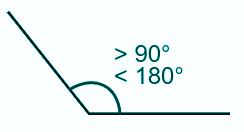Let us consider the below example,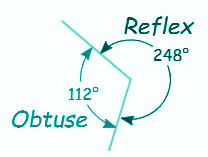From the figure, it is noted that the smaller angle is called the obtuse angle, and the larger angle formed is called the reflex angle. Thus, the degree of the obtuse angle is more than 90 degrees and less than the straight angle.

## Obtuse Angle of a Triangle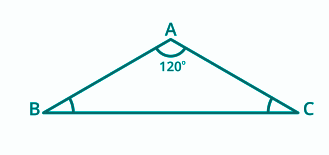The obtuse angle of a triangle is a triangle, where one of its angles of a triangle is greater than 90 degrees. An obtuse triangle may be an isosceles or scalene triangle. An equilateral triangle cannot be obtuse. The angle opposite to the obtuse angle is the longest side of the triangle. Similarly, a triangle cannot have a right angle and obtuse angle at the same time. If one of the angles of a triangle is obtuse, the other two angles of a triangle must be acute.

## Obtuse Angle of a Rhombus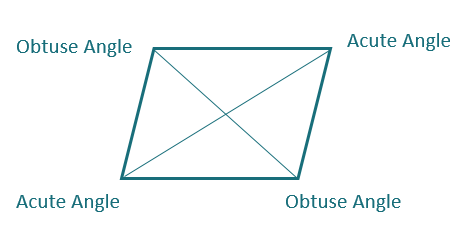A rhombus is a quadrilateral which has four sides. The opposite angles of a rhombus are congruent to each other. A rhombus has two congruent opposite acute angles and two congruent opposite obtuse angles. The sum of two consecutive internal angles is supplementary. It means that if an acute angle and the obtuse angle is added, its sum is 180 degrees.

## Obtuse Angle of a Parallelogram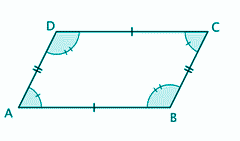The opposite angles of a parallelogram are parallel to each other. A Parallelogram has parallel sides. It means that two angles will be acute and two angles will be obtuse. Since the parallelogram leans over, the angle measure of the obtuse angle will be greater, and the measure of acute angle will be smaller. Here, ∠A and ∠C are the acute angles, whereas ∠B and ∠D are the obtuse angles.

### Obtuse Angle Examples

The following are the examples of the obtuse angles. Go through the below problems to learn about the obtuse angles.

Example 1:

Find the value of x for the given triangle ABC.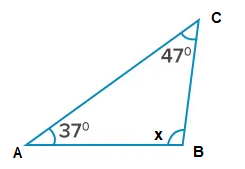Solution:

For the triangle ABC, the two given angle measures are:

∠A = 37° and ∠C = 47°

We know that the sum of the interior angles of a triangle is 180 degrees.

Therefore,

∠A + ∠B + ∠C = 180° …..(1)

Now,substitute the values ∠A = 37° and ∠C = 47° in (1)

37° + ∠B + 47° = 180°

∠B + 84° = 180°

∠B = 180° – 84°

∠B = 96°.

Thus, the value of x is 96°, which is an obtuse angle.

Example 2:

If the reflex angle of the given rays is 244°, then what will be the measure of the interior angle?

Solution:

Given that, the measure of the reflex angle = 244°

To find the unknown interior angle, use the relation:

Reflex angle + Unknown angle = 360° …. (1)

Let the unknown angle be “x”.

Now, substitute the value of the reflex angle in (2)

244° + x = 360°

x = 360° – 244°

x = 116°

Hence, the unknown angle obtained is an obtuse angle which measures 116°.

Register with BYJU’S – The Learning App and download the app today to explore more videos.

## Frequently Asked Questions on Obtuse Angle – FAQs

### What are the six types of angles?

The six types of angles formed in the plane surface are:
Acute Angle
Obtuse Angle
Right Angle
Straight Angle
Reflex Angle
Full Angle

### What is the shape of an obtuse angle?

As we know, the measure of an obtuse angle is greater than 90 degrees. We can observe many things in our life which are in the shape of obtuse angles such as hangars used to keep clothes in cupboards, hour hand and minute hand of a clock at 4 O’ clock and so on.

### What is a zero angle?

If an angle has a measure of zero degrees, then it is called a zero angle. In other words, when two rays coincide with each other, then the angle between these two rays is zero angle.

### What type of angle is 200 degrees?

200 degrees is greater than 180 degrees; therefore, it is a reflex angle. Since reflex angles are the types of angles whose measurement is more than 180 degrees but less than 360 degrees.

### What type of angle is 180 degrees?

Any angle which measures exactly 180 degrees is called a straight angle. Also, when the angle between two lines is 180 degrees, then they are called the linear pair of angles, i.e. they form a straight line.

Quiz on Obtuse angle